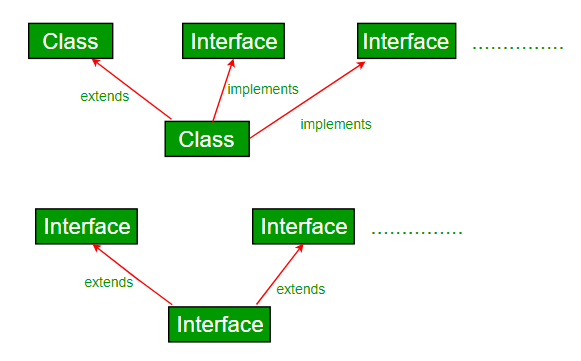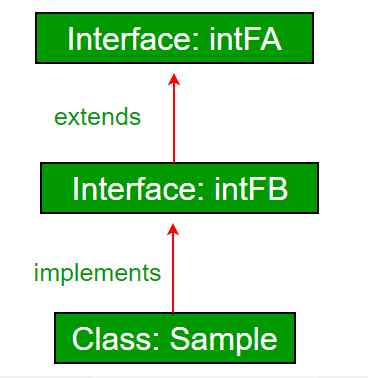# Interfaces and Inheritance in Java

A class can extend another class and can implement one and more than one Java interface. Also, this topic has a major influence on the concept of Java and Multiple Inheritance.Note: This Hierarchy will be followed in the same way, we cannot reverse the hierarchy while inheritance in Java. This means that we cannot implement a class from the interface because Interface-to-Class inheritance is not allowed, and it goes against the fundamental principles of class-based inheritance. This will also breach the relationship known as the Is-A relationship, where a subclass is a more specialized version of its superclass.

Example:

## Java

 `// Java program to demonstrate that a class can` `// implement multiple interfaces` `import` `java.io.*;`   `interface` `intfA {` `    ``void` `m1();` `}`   `interface` `intfB {` `    ``void` `m2();` `}`   `// class implements both interfaces` `// and provides implementation to the method.` `class` `sample ``implements` `intfA, intfB {` `    ``@Override` `public` `void` `m1()` `    ``{` `        ``System.out.println(``"Welcome: inside the method m1"``);` `    ``}`   `    ``@Override` `public` `void` `m2()` `    ``{` `        ``System.out.println(``"Welcome: inside the method m2"``);` `    ``}` `}`   `class` `GFG {` `    ``public` `static` `void` `main(String[] args)` `    ``{` `        ``sample ob1 = ``new` `sample();`   `        ``// calling the method implemented` `        ``// within the class.` `        ``ob1.m1();` `        ``ob1.m2();` `    ``}` `}`

Output:

```Welcome: inside the method m1
Welcome: inside the method m2

```

Interface inheritance: An Interface can extend another interface.Inheritance is inheriting the properties of the parent class into the child class.

1. Inheritance in Java is a mechanism in which one object acquires all the properties and behaviors of a parent object.
2. The idea behind inheritance in Java is that you can create new classes that are built upon existing classes. When you inherit from an existing class, you can reuse methods and fields of the parent class.
3. You can also add new methods and fields in your current class.
4. Inheritance represents the IS-A relationship which is also known as the parent-child relationship.

#### Example

```Dog IS_A Animal
Car IS_A Vehicle
Employee IS_A Person
Surgeon IS_A Doctor etc.
```

Below is the implementation of the above method:

## Java

 `// Animal is a Parent class` `class` `Animal {` `    ``public` `void` `eat()` `    ``{` `        ``System.out.println(``"Animal is eating"``);` `    ``}` `}`   `// Here Dog is derived from Animal class` `class` `Dog ``extends` `Animal {` `    ``public` `static` `void` `main(String args[])` `    ``{` `        ``// creating object of Dog class` `        ``Dog d = ``new` `Dog();`   `        ``// Now, Dog can access eat() method of Animal class` `        ``d.eat();` `    ``}` `}`

Output

```Animal is eating

```

### Syntax of Java Inheritance

```class <Subclass-name> extends <Superclass-name>
{
//methods and fields
}```

Note: The extends keyword indicates that you are making a new class that derives from an existing class. The meaning of “extends” is to increase the functionality.

## Example of Java Inheritance

Below is the implementation of Java Inheritance:

## Java

 `// Java Program to demonstrate ` `// Java Inheritance`   `// Parent Class` `class` `Person1 {` `      ``// Variables` `    ``int` `id;` `    ``String name;` `  `  `      ``// Java Methods` `    ``void` `set_Person(``int` `id, String name)` `    ``{` `        ``try` `{` `            ``this``.id = id;` `            ``this``.name = name;` `        ``}` `        ``catch` `(Exception ex) {` `            ``ex.printStackTrace();` `        ``}` `    ``}` `    ``void` `disp_Person()` `    ``{` `        ``System.out.print(id + ``"\t"` `+ name + ``"\t"``);` `    ``}` `}`   `// Child Class` `class` `Employee1 ``extends` `Person1 {` `    ``int` `sal;` `    ``String desgn;` `    ``void` `set_Emp(``int` `id, String name, String desgn, ``int` `sal)` `    ``{` `        ``try` `{` `            ``set_Person(id, name);` `            ``this``.desgn = desgn;` `            ``this``.sal = sal;` `        ``}` `        ``catch` `(Exception ex) {` `            ``ex.printStackTrace();` `        ``}` `    ``}` `    ``void` `disp_Emp()` `    ``{` `        ``disp_Person();` `        ``System.out.print(desgn + ``"\t"` `+ sal);` `    ``}` `  `  `      ``// Main function` `    ``public` `static` `void` `main(String args[])` `    ``{`   `        ``Employee1 e1 = ``new` `Employee1();` `        ``e1.set_Emp(``1001``, ``"Manjeet"``, ``"AP"``, ``20000``);` `        ``e1.disp_Emp();` `    ``}` `}`

Output

```1001    Manjeet    AP    20000
```

## Types of inheritance in Java

1. Java supports three types of inheritance in Java: single-level, multilevel, and hierarchical inheritance in the case of classes to avoid ambiguity.
2. In Java programming, multiple and hybrid inheritance is supported through the interface only.

### 1. Single Inheritance Example

When a class inherits another class, it is known as a single inheritance.

## Java

 `// Single-Level inheritance` `// Class B ---> Class A, which means that class B is derived from Class A`   `class` `A {` `    ``int` `a;` `    ``void` `set_A(``int` `x) { ` `      ``a = x; ` `    ``}` `}`   `// Class B have access to all public and protected methods and data members of Class A` `class` `B ``extends` `A {` `    ``int` `b, product;` `    ``void` `set_B(``int` `x) { ` `      ``b = x; ` `    ``}` `    ``void` `cal_Product()` `    ``{` `        ``product = a * b;` `        ``System.out.println(``"Product = "` `+ product);` `    ``}` `    ``public` `static` `void` `main(String[] args)` `    ``{` `        ``B b = ``new` `B();` `        ``b.set_A(``5``);` `        ``b.set_B(``5``);` `        ``b.cal_Product();` `    ``}` `}`

Output

```Product = 25

```

### 2. Multilevel Inheritance Example

When there is a chain of inheritance, it is known as multilevel inheritance.

Below is the implementation of the above method:

## Java

 `// Multilevel inheritance` `// Class C ---> Class B ---> Class A` `// Class C is derived from Class B which in correspondence derived from Class A`   `class` `A {` `    ``int` `a;` `    ``void` `set_A(``int` `x) { ` `      ``a = x; ` `    ``}` `}`   `// Child of Class A` `class` `B ``extends` `A {` `    ``int` `b;` `    ``void` `set_B(``int` `x) { ` `      ``b = x; ` `    ``}` `}`   `// Child of Class B but have access to methods of both classes, i.e., Class A and B` `class` `C ``extends` `B {` `    ``int` `c, product;` `    ``void` `cal_Product()` `    ``{` `        ``product = a * b;` `        ``System.out.println(``"Product = "` `+ product);` `    ``}` `    ``public` `static` `void` `main(String[] args)` `    ``{` `        ``C c = ``new` `C();` `          ``// Class C accesses methods of both class A and B` `        ``c.set_A(``5``);` `        ``c.set_B(``5``);` `        ``c.cal_Product();` `    ``}` `}`

Output

```Product = 25

```

### 3. Hierarchical Inheritance Example

When two or more classes inherit a single class, it is known as hierarchical inheritance.

Below is the implementation of the mentioned topic:

## Java

 `// Hierarchical inheritance` `// Class C ---> Class A <--- Class B` `// Both Class B and C inherits Class A`   `class` `A {` `    ``int` `a;` `    ``void` `set_A(``int` `x) { ` `          ``a = x; ` `          ``System.out.println(``"Setting A's value to = "` `+ x);` `    ``}` `}`   `// Class B derived from Class A` `class` `B ``extends` `A {` `    ``int` `b;` `    ``void` `set_B(``int` `x) { ` `      ``b = x; ` `      ``System.out.println(``"Setting B's value to = "` `+ b);` `    ``}` `}`   `// Class C also derived from Class A` `class` `C ``extends` `A {` `    ``int` `c;` `    ``void` `set_C(``int` `x) {` `      ``c = x;` `      ``System.out.println(``"Setting C's value to = "` `+ c);` `    ``}` `}`   `public` `class` `GFG {` `      ``public` `static` `void` `main(String[] args) {` `          ``C c = ``new` `C();` `          ``c.set_C(``5``);` `          ``c.set_A(``50``);` `          `  `          ``B b = ``new` `B();` `          ``b.set_B(``10``);` `          ``b.set_A(``15``);` `    ``}` `}`

Output

```Setting C's value to = 5
Setting A's value to = 50
Setting B's value to = 10
Setting A's value to = 15

```

## Java

 `// Java program to demonstrate inheritance in` `// interfaces.` `import` `java.io.*;` `interface` `intfA {` `    ``void` `geekName();` `}`   `interface` `intfB ``extends` `intfA {` `    ``void` `geekInstitute();` `}`   `// class implements both interfaces and provides` `// implementation to the method.` `class` `sample ``implements` `intfB {` `    ``@Override` `public` `void` `geekName()` `    ``{` `        ``System.out.println(``"Rohit"``);` `    ``}`   `    ``@Override` `public` `void` `geekInstitute()` `    ``{` `        ``System.out.println(``"JIIT"``);` `    ``}`   `    ``public` `static` `void` `main(String[] args)` `    ``{` `        ``sample ob1 = ``new` `sample();`   `        ``// calling the method implemented` `        ``// within the class.` `        ``ob1.geekName();` `        ``ob1.geekInstitute();` `    ``}` `}`

Output:

```Rohit
JIIT
```

An interface can also extend multiple interfaces.

## Java

 `// Java program to demonstrate multiple inheritance` `// in interfaces`   `import` `java.io.*;`   `interface` `intfA {` `    ``void` `geekName();` `}`   `interface` `intfB {` `    ``void` `geekInstitute();` `}`   `// always remember that interfaces always extends interface ` `// but a class always implements a interface` `interface` `intfC ``extends` `intfA, intfB {` `    ``void` `geekBranch();` `}`   `// class implements both interfaces and provides` `// implementation to the method.` `class` `sample ``implements` `intfC {` `    ``public` `void` `geekName() { System.out.println(``"Rohit"``); }`   `    ``public` `void` `geekInstitute()` `    ``{` `        ``System.out.println(``"JIIT"``);` `    ``}`   `    ``public` `void` `geekBranch() { System.out.println(``"CSE"``); }`   `    ``public` `static` `void` `main(String[] args)` `    ``{` `        ``sample ob1 = ``new` `sample();`   `        ``// calling the method implemented` `        ``// within the class.` `        ``ob1.geekName();` `        ``ob1.geekInstitute();` `        ``ob1.geekBranch();` `    ``}` `}`

Output

```Rohit
JIIT
CSE

```

### Q. Why Multiple Inheritance is not supported through a class in Java, but it can be possible through the interface?

Multiple Inheritance is not supported by class because of ambiguity. In the case of interface, there is no ambiguity because the implementation of the method(s) is provided by the implementing class up to Java 7. From Java 8, interfaces also have implementations of methods. So if a class implements two or more interfaces having the same method signature with implementation, it is mandated to implement the method in class also.

Refer to Java and Multiple Inheritance for details.

Feeling lost in the vast world of Backend Development? It's time for a change! Join our Java Backend Development - Live Course and embark on an exciting journey to master backend development efficiently and on schedule.
What We Offer:
• Comprehensive Course
• Expert Guidance for Efficient Learning
• Hands-on Experience with Real-world Projects
• Proven Track Record with 100,000+ Successful Geeks

Previous
Next